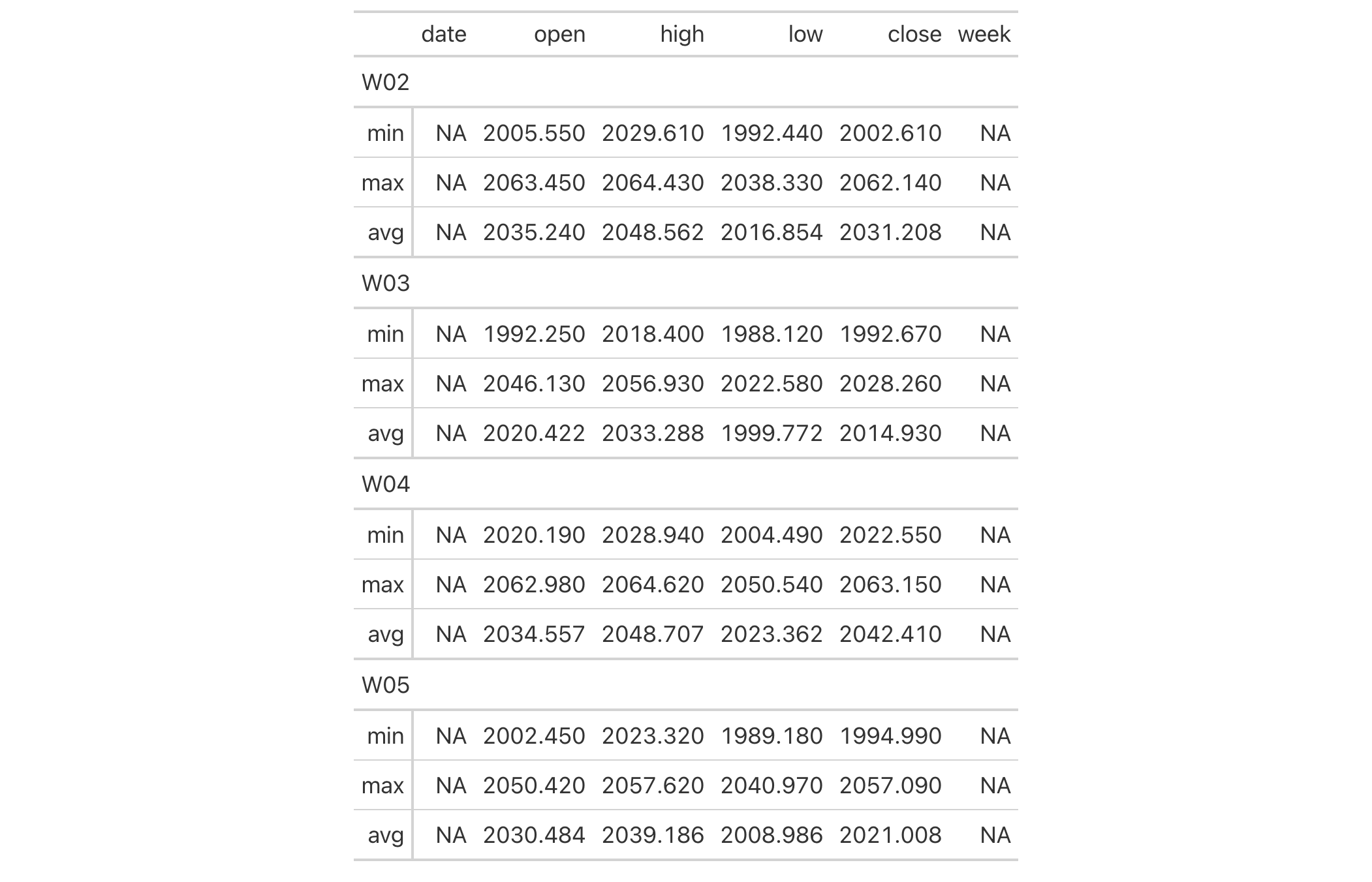Get a list of summary row data frames from a gt_tbl object where summary rows were added via the summary_rows() function. The output data frames contain the group_id and rowname columns, whereby rowname contains descriptive stub labels for the summary rows.

## Usage

extract_summary(data)

## Arguments

data

A table object that is created using the gt() function.

## Value

A list of data frames containing summary data.

## Examples

Use sp500 to create a gt table with row groups. Create summary rows labeled as min, max, and avg for every row group with summary_rows(). Then, extract the summary rows as a list object.

summary_extracted <-
sp500 %>%
dplyr::filter(date >= "2015-01-05" & date <="2015-01-30") %>%
dplyr::arrange(date) %>%
dplyr::mutate(week = paste0("W", strftime(date, format = "%V"))) %>%
gt(
rowname_col = "date",
groupname_col = "week"
) %>%
summary_rows(
groups = TRUE,
columns = c(open, high, low, close),
fns = list(
min = ~min(.),
max = ~max(.),
avg = ~mean(.)
),
formatter = fmt_number,
use_seps = FALSE
) %>%
extract_summary()

summary_extracted

Use the summary list to make a new gt table. The key thing is to use dplyr::bind_rows() and then pass the tibble to gt().

summary_extracted %>%
unlist(recursive = FALSE) %>%
dplyr::bind_rows() %>%
gt(groupname_col = "group_id")## Function ID

13-5

Other Export Functions: as_latex(), as_raw_html(), as_rtf(), gtsave()